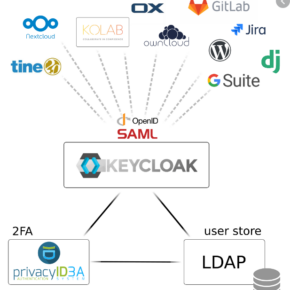autonomic-cooperative
/
keycloak-collective-portal
Community Keycloak SSO user management.
You can not select more than 25 topics Topics must start with a letter or number, can include dashes ('-') and can be up to 35 characters long.

#### 44 lines 976 B Raw Permalink Blame History

 `[tool.poetry]` `name = "keycloak-collective-portal"` `version = "0.1.0"` `description = "Community Keycloak SSO user management."` `authors = ["decentral1se "]` `license = "AGPLv3"` ``` ``` `[tool.poetry.dependencies]` `python = "^3.9"` `fastapi = "^0.65.2"` `uvicorn = {extras = ["standard"], version = "^0.14.0"}` `Jinja2 = "^3.0.1"` `itsdangerous = "^2.0.1"` `Authlib = "^0.15.4"` `httpx = "^0.18.1"` `aioredis = "^1.3.1"` `humanize = "^3.7.1"` `python-multipart = "^0.0.5"` `python-keycloak = "^0.25.0"` `aiofiles = "^0.7.0"` `email-validator = "^1.1.3"` ``` ``` `[tool.poetry.dev-dependencies]` `black = "^21.6b0"` `flake8 = "^3.9.2"` `isort = "^5.8.0"` `mypy = "^0.902"` ``` ``` `[tool.black]` `line-length = 80` `target-version = ["py39"]` `include = '\.pyi?\$'` ``` ``` `[tool.isort]` `include_trailing_comma = true` `known_first_party = "keycloak_collective_portal"` `known_third_party = "pytest"` `line_length = 80` `multi_line_output = 3` `skip = ".tox"` ``` ``` `[build-system]` `requires = ["poetry-core>=1.0.0"]` ```build-backend = "poetry.core.masonry.api" ``` ``` ```